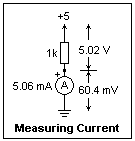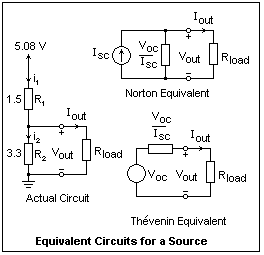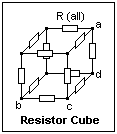## Measuring Currents

How to measure currents, and how to characterize power sources

If you now set your meter aside, and later pick it up to measure a voltage, you may not notice that the meter is set to current, and that the lead is plugged into the ΩA jack. When you try to measure the voltage, you will get zero. Noticing that the selector is set to current, you will now move it to voltage--but still get zero. Oh yes, the lead should be plugged into the + jack. When you do this, the reading is still zero. What has happened is that you blew the protective fuse the very first time, so you still have a healthy meter, but it needs a new fuse. As long as you leave the lead in the + jack, this cannot happen, and you are safe. If the lead is in ΩA, and the selector is in either current or resistance function, a fuse will blow to protect the meter as soon as you touch the leads across a voltage. The safest idea of all is to leave the lead in the + jack at all times and only measure voltages. If you have to measure a resistance or a current, make a habit of always moving back to the + jack afterwards, or else buy a lot of fuses.

There is another way to measure currents that is utterly safe and convenient, and very practical in electronics. Most currents in electronics pass through resistances whose value is marked and obvious, and the current can be obtained from Ohm's law, I = V/R. Usually, it is convenient to measure I in mA, V in volts, and R in kiloohms. V is the voltage across the resistor, and I is the current through it. The fact that we often measure voltages with respect to some common point (ground or earth) should not lead you to ignore that voltages are always between two points, never just at a point. Also, both currents and voltages have direction, for which some reference direction that is called positive is arbitrarily assumed. The opposite direction is then indicated by a minus sign. The reference direction is completely arbitrary, though one generally makes a choice to get as few negative signs as possible. The fact that no sign is used instead of an explicit + should not lead you to ignore the fact that there is always a sign, + or -.

Collect some 1/4 W resistors of values between 1k and 10k (k = kΩ), and note the color codes. The usual 5% resistors are fine, but you can stick in some 1% resistors if you want. Measure their resistances, and note that the values are rather close to the nominal values given by the color code. This was not always the case in the past, but now manufacturing methods produce resistors of precise values, instead of a continuous range. It will always be sufficent in electronics to assume that the resistance of a resistor is the marked nominal value. In the examples, I will use the values and readings that I obtained myself, which you should replace by the values and readings that you make.Let's first measure the current through a resistor, using the two principal methods available to us. I'll suppose that you have two meters available, which will give us a bonus, but only one meter will do. Connect a 1k resistor from the +5 row in a breadboard to some hole below it, the red lead of the meter to this end of the resistor, and the black lead to the G (ground or earth) row of the breadboard. The circuit is shown in the diagram on the right, with my actual measurements shown. Yours will be a little different. The red lead should be in the ΩA jack, and the selector should be to DC current, 2 mA range (or as appropriate for your meter). Turn on the power, and read the current, which should be about +5.0 mA. It is very seldom necessary to take readings to a greater accuracy than two digits in electronics, but you can include a third digit as a guard, rounding off later. Now take another meter, set to DC volts, 20 V range (or as appropriate), and connect its leads to the ends of the resistor. If you have only one meter, connect the lower end of the resistor to the G row. You will get about +5.0 V (in my case, 5.08 V), which divided by 1k gives 5 mA. In the figure, 5.02 is about the same as 5.06, and trying to make them exactly the same is not worth the effort. We are using different meters, and the resistance is not exactly 1.000k anyway. It's comforting for you to see that the two methods give the same result, so don't omit the experiment. Otherwise, your unconscious will always have a doubt.

If you have two meters, use the second meter to measure not only the voltage across the resistor, but also the voltage across the other meter. It will not be zero, but will be in the millivolt range. My meter gave 60 mV, on the 2 mA scale. This is much less than the 5 V applied to the resistor, but it is not zero. In my case, it was equivalent to a resistance of 12 Ω, compared to the resistance of 1000 Ω in series with it. The actual value is not of much importance, except that its presence should be recognized, and that the insertion of the meter has a disturbing effect on the circuit, here about 1%. In the voltmeter method of determining the current, there is a similar effect due to the shunting of some of the current through the meter. In my case, the meter has a resistance of some 10M (M = megohm), which is in parallel with the 1k resistor. The effect on the circuit is only 0.1% in this case. However, if the resistor were a 1M resistor, the disturbance would amount to 10%. The effect of the meter on the circuit should always be remembered. A digital multimeter disturbs the typical electronic circuit with resistances of 100k and below very little, but watch out when the resistances are high and the currents low.A simple series-parallel circuit is shown in the figure. I have labeled the resistors the usual way, and chosen the postitive directions of the currents and labeled them. The values of the resistors are given with the convention that a value with a decimal point is assumed to by kiloohms, with no decimal point, simply ohms.The currents will all turn out positive, but this is only a convenience. It is easy to find the currents in any such circuit by combining resistors in series and parallel, and you probably know how to do this already. The parallel combination of the two lower resistors is equivalent to 1.14k, so i1 = 5.08 / 2.14 = 2.37 mA. This current divides in the lower resistors in inverse ratio to the resistances, so i2 = 2.37 (1.5/6.2) = 0.57 mA, and i3 = 2.37 (4.7/6.2) = 1.80 mA, using the current divider rule. The voltage across the upper resistor is 5.08 (1.0/2.14) = 2.37 V, and the voltage across the lower resistors is 5.08 (1.14/2.14) = 2.71 V, by the voltage divider rule. All such circuits can be solved by such means, but usually you do not have to do this, unless you enjoy doing it.

There is a node marked "a" in this circuit. We know that the sum of the currents flowing into this node is zero, and indeed 2.37 - 0.57 - 1.80 = 0, for the currents we have just found. If we knew the voltage at node "a" with respect to ground, we would then know the voltages across each of the resistors, and could find the currents by Ohm's Law. In fact, we could take the voltage of node a as an unknown, and solve the equation we get from equating the sum of the currents to zero for this unknown voltage, and then find all the currents. This is the nodal method of solution, which you learned in your Circuits course. We have an easier way--simply use the digital multimeter to measure this voltage directly. Of course, the result is 2.70 V, from which we can find all the currents immediately, with no need for algebra. It's curious that the Circuits course is a course in learning how to do what you will never need to do! In general, we measure the node voltages (with respect to ground) and determine all the currents from them, in any practical circuit. This is also the fundamental way to find out if a circuit is working as intended. Simply measure the node voltages and compare them with the normal values.The circuit in the figure cannot be solved as a series-parallel circuit. If we had to solve it, the two unknown node voltages can be taken as unknowns, two nodal equations written, and the two unknowns found. Only a professor would use two loop currents, only a sophomore five unknown currents. If you have just two unknowns, there is some hope of getting a correct solution. Humans cannot work out determinants of order 3 and greater without error, so we never do this. Wire up the circuit, and measure the node voltages. For my circuit, Va = 3.21 V, and Vb = 2.29 V, from which all five currents can be found at once. I get 1.87, 0.97, 0.59, 1.53 and 0.92 mA. Check that the net current flowing into each node is zero; this will pick up any errors in finding the currents.

Now there is something very important to learn about power sources. We have been using the +5 regulated supply on the breadboard. Within limits, it supplies very close to 5 volts no matter what we connect to it, or, in other words, no matter how much current we draw from it. It is, in fact, a pretty good voltage source, as are batteries and the 115 V AC supply. We know that it is not nice to connect a low resistance across its output, since I = V/R, and low R means high I. Our 5 V supply is nicely protected; it will give us a certain amount of current, and then no more, reducing its voltage as necessary to limit the current. The AC supply will give a zap and a blown fuse--it's also protected. The battery will give a current depending on its construction--a lead-acid car battery is supposed to give a large current on near short-circuit, and a flashlight bulb is a pretty low resistance, too. In any case, the power output will be E2/R, so if R is small, there will be some heat somewhere!In electronics, we often have power sources and their loads, with more complicated characteristics than DC power supplies and batteries. Build a source by connecting a voltage divider between +5 and G on the breadboard, as shown in the figure, taking the output from the node between the resistors. With no load (infinite resistance) the voltage at the output node is 5.08 (3.3/4.8) = 3.49 V. This is the open circuit output voltage, VOC. Now connect resistances of 10k, 4.7k and 1.0k successively to the output node, and measure the voltage. Finally, connect a short circuit. If you want, use the current function of the digital multimeter. The short circuit output current ISC of about 3.4 mA won't blow any fuses. With a source like this one, you can connect either an open circuit or a short circuit with no damage. The normal effect of a decreasing output voltage with an increasing output current is observed.

Now plot the output voltage against the output current. An accurate sketch will be sufficient to show you the result. The plot is a straight line connecting VOC on the vertical axis with ISC on the horizontal axis. Of course the line is straight, because resistors are linear circuit elements, and this is just what is meant by that name. The slope of the line is VOC/ISC, a resistance called the Thévenin or internal resistance of the source. In this example, it is about 1.03k. In the figure are shown two other circuits that would give exactly the same output voltage and current as the actual source. The Thévenin equivalent has an ideal voltage source equal to VOC, while the Norton equivalent has an ideal current source equal to ISC. With the example source, it is practical to measure each of these quantities directly. Usually, this is not practical. In the case of the AC power line, or a lead-acid battery, ISC is dangerously large, and the low internal resistance of these sources will allow it to flow. In this case, it is necessary to use some reasonable resistance instead of a short circuit, and note the drop in output voltage, which will be small. From this the internal resistance can be found. For example, if the output voltage drops by 2.8 V when a current of 0.7 A is drawn, then the internal resistance is 2.8 / 0.7 = 4.0 Ω.

The compliance of a current source is the range of voltages it can place across a load. A stiff current source is one with a large Norton resistance, so the current that it supplies is nearly constant.

Use the Thévenin equivalent of the example source to find the output voltages for each of the load resistances. Simply use the voltage divider rule in each case, which is easier than solving the actual circuit, even in this simple case. With practical electronic sources, the saving in work is dramatic. The currents can be found in the same way by using the Norton equivalent.

It is well to observe that the currents and voltages inside the equivalent circuits are not those found in the actual circuit, where conditions can be quite different. The equivalent circuits are for use only to find the current and voltage supplied to the load, not for finding anything out about the source, and certainly not for any power calculations. For example, if the load for the example source is a short circuit, then the actual power dissipated in the source, in the 1.5k resistor, is 17.2 mW, but in the Thévenin equivalent, it would be only 11.5 mW. In the Norton equivalent, it would be zero! The internal resistance does not exist as an actual resistance (unless the actual circuit is identical to the equivalent circuit), and does not dissipate actual power.

The usual voltage source has a small internal resistance, so the plot of voltage versus current is nearly horizontal, and is used near the vertical axis, where the voltage is roughly the open circuit voltage. The short circuit current is well out of the normal range of operation, and would be quite large if the source did not destroy itself in trying to reach it. Such a source must never be short-circuited. The usual current source has a large internal resistance, so the plot of voltage versus current is nearly vertical, and is used near the horizontal axis, where the current is roughly the short-circuit current. The open circuit voltage is well out of the normal range of operation, and would be very high if the source did not destroy itself in trying to reach it. Such a source must never be open-circuited. Voltage sources are quite common in most applications of electricity, but in electronics current sources are also common.

### An ExerciseThe three-dimensional circuit at the right is a good test of your reasoning with respect to circuits. All the resistances are equal, so symmetry can be used to analyze the circuit. Find the resistance between node "a" and node "b" at the ends of a body diagonal of the cube. Now find the resistance between node "a" and node "c" across a face diagonal, and finally the resistance from node "a" to node "d" along an edge. The first is rather easy, the second harder, and the third the most difficult. In every case, there is no need to use determinants or loop currents, or any other excessive complication, just series-parallel circuit analysis helped by symmetry. The answers are: 5R/6, 3R/4 and 7R/12. Solder 12 resistors together to make a cube and test these results.